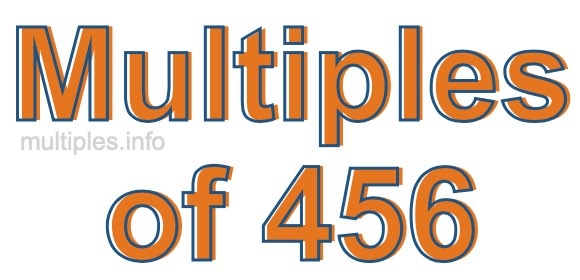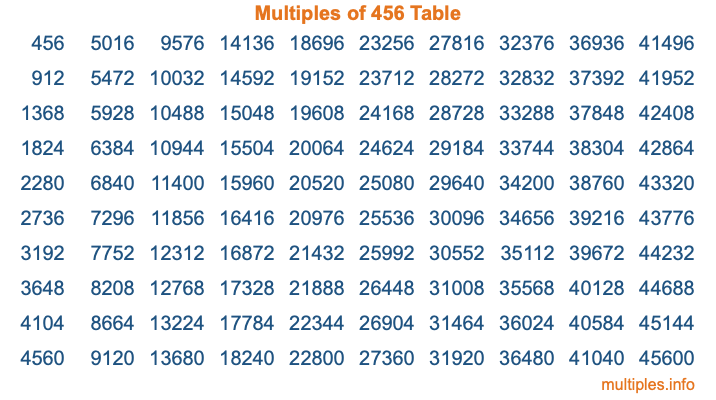Multiples of 456Welcome to the Multiples of 456 page. Here we will first teach you everything you will ever need to know about the multiples of 456, and then give you a study guide summary of everything we taught you to make sure you remember it all. Use this page to look up facts and learn information about the multiples of 456. This page will make you a multiples of four hundred fifty-six expert!

Definition of Multiples of 456
Multiples of 456 are all the numbers that when divided by 456 equal an integer. Each of the multiples of 456 are called a multiple. A multiple of 456 is created by multiplying 456 by an integer.

Therefore, to create a list of multiples of 456, you start with 1 multiplied by 456, then 2 multiplied by 456, then 3 multiplied by 456, and so on for as long as you want. Thus, the list of the first five multiples of 456 is 456, 912, 1368, 1824, and 2280. To see a larger list of multiples of 456, see the printable image of Multiples of 456 further down on this page. We also have a category where you can choose any nth multiple of 456.

Multiples of 456 Checker
The Multiples of 456 Checker below checks to see if any number of your choice is a multiple of 456. In other words, it checks to see if there is any number (integer) that when multiplied by 456 will equal your number. To do that, we divide your number by 456. If the the quotient is an integer, then your number is a multiple of 456.

Is  a multiple of 456?

Least Common Multiple of 456 and ...
A Least Common Multiple (LCM) is the lowest multiple that two or more numbers have in common. This is also called the smallest common multiple or lowest common multiple and is useful to know when you are adding our subtracting fractions. Enter one or more numbers below (456 is already entered) to find the LCM.

Check out our LCM Calculator if you need more details about the Least Common Multiple or if you need the LCM for different numbers for adding and subtraction fractions.

nth Multiple of 456
As we stated above, 456 is the first multiple of 456, 912 is the second multiple of 456, 1368 is the third multiple of 456, and so on. Enter a number below to find the nth multiple of 456.

th multiple of 456

Multiples of 456 vs Factors of 456
456 is a multiple of 456 and a factor of 456, but that is where the similarities end. All postive multiples of 456 are 456 or greater than 456. All positive factors of 456 are 456 or less than 456.

Below is the beginning list of multiples of 456 and the factors of 456 so you can compare:

Multiples of 456: 456, 912, 1368, 1824, 2280, etc.

Factors of 456: 1, 2, 3, 4, 6, 8, 12, 19, 24, 38, 57, 76, 114, 152, 228, 456

As you can see, the multiples of 456 are all the numbers that you can divide by 456 to get a whole number. The factors of 456, on the other hand, are all the whole numbers that you can multiply by another whole number to get 456.

It's also interesting to note that if a number (x) is a factor of 456, then 456 will also be a multiple of that number (x).

Multiples of 456 vs Divisors of 456
The divisors of 456 are all the integers that 456 can be divided by evenly. Below is a list of the divisors of 456.

Divisors of 456: 1, 2, 3, 4, 6, 8, 12, 19, 24, 38, 57, 76, 114, 152, 228, 456

The interesting thing to note here is that if you take any multiple of 456 and divide it by a divisor of 456, you will see that the quotient is an integer.

Multiples of 456 Table
Below is an image of the first 100 multiples of 456 in a table. The table is in chronological order, column by column. The first column has the first ten multiples of 456, the second column has the next ten multiples of 456, and so on.The Multiples of 456 Table is also referred to as the 456 Times Table or Times Table of 456. You are welcome to print out our table for your studies.

Negative Multiples of 456
Although not often discussed or needed in math, it is worth mentioning that you can make a list of negative multiples of 456 by multiplying 456 by -1, then by -2, then by -3, and so on, to get the following list of negative multiples of 456:

-456, -912, -1368, -1824, -2280, etc.

Multiples of 456 Summary
Below is a summary of important Multiples of 456 facts that we have discussed on this page. To retain the knowledge on this page, we recommend that you read through the summary and explain to yourself or a study partner why they hold true.

There are an infinite number of multiples of 456.

A multiple of 456 divided by 456 will equal a whole number.

456 divided by a factor of 456 equals a divisor of 456.

The nth multiple of 456 is n times 456.

The largest factor of 456 is equal to the first positive multiple of 456.

456 is a multiple of every factor of 456.

456 is a multiple of 456.

A multiple of 456 divided by a divisor of 456 equals an integer.

456 divided by a divisor of 456 equals a factor of 456.

Any integer times 456 will equal a multiple of 456.

Multiples of a Number
Here you can get the multiples of another number, all with the same attention to detail as we did for multiples of 456 on this page.

Multiples of
Multiples of 457
Did you find our page about multiples of four hundred fifty-six educational? Do you want more knowledge? Check out the multiples of the next number on our list!